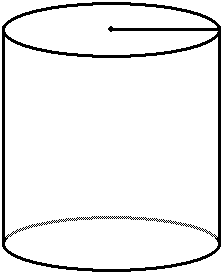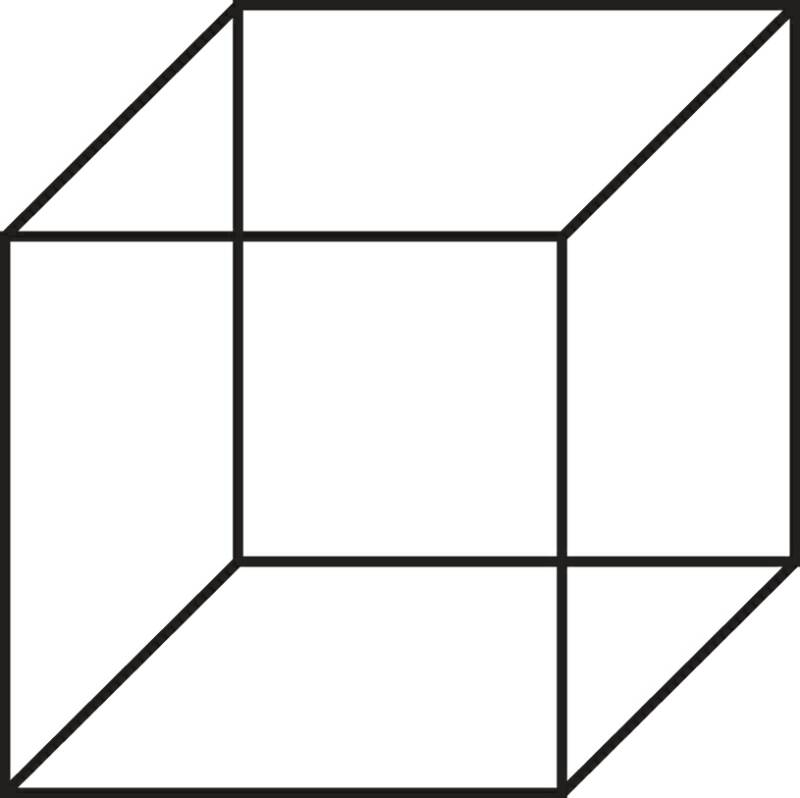VOLUME  FORMULASCone:
(dirt stockpiles)Volume = (pi xx h)/3
pi = 3.1415
h = heightSphere:Volume = (4 x pi x r³)/3
pi = 3.1415

Call me today  678-878-0641  or email me
DirtEstimator@div2est.com

AREA  FORMULASCircle:Area  = pi x
pi = 3.1415
h is the heightTriangle:Area  = b x h x 1/2
b = base
h = heightEllipse:Area  = pi x r  x r
pi = 3.1415
r1 = radius of the long axis
r2 = radius of the short axisThere are certain formulas anyone who works in grading / excavating / pipe laying will need to know.  Some will be used everyday, others not so often.  All should be committed to memory.  If that is not possible, they should be kept in a handy place.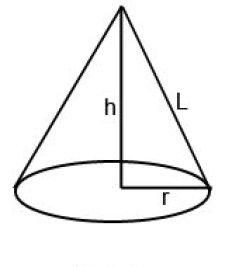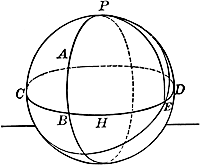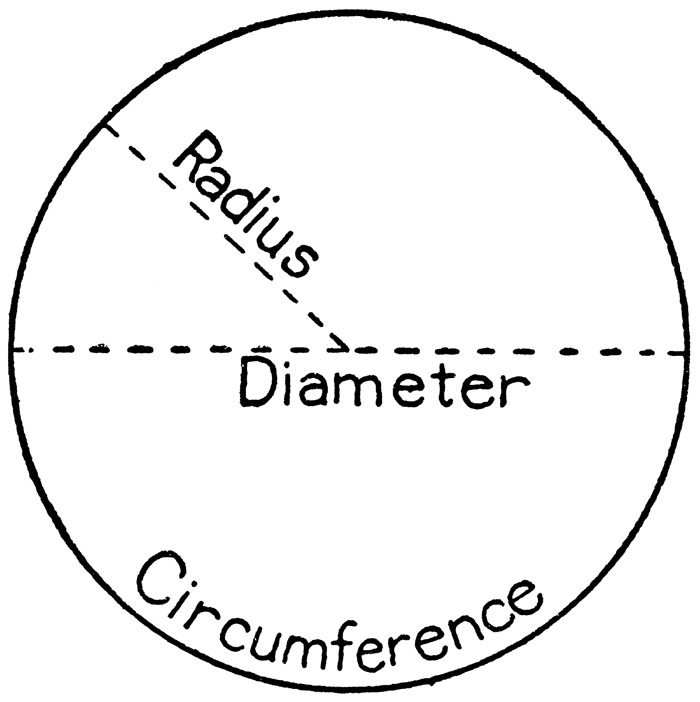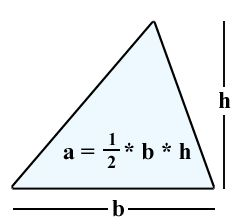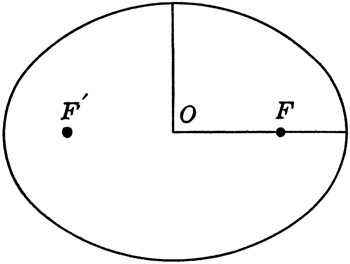1
2VOLUME  FORMULASCube:Volume = a³ = a x a x aCylinder:
(manholes & pipe)Volume = pi xx h
pi = 3.1415
h = heightRectangle:
(Box Culverts,
Trenches, Slabs)Volume = l x w x h
l = the length
w = width
h = height

Call me today  678-878-0641  or email me
DirtEstimator@div2est.com